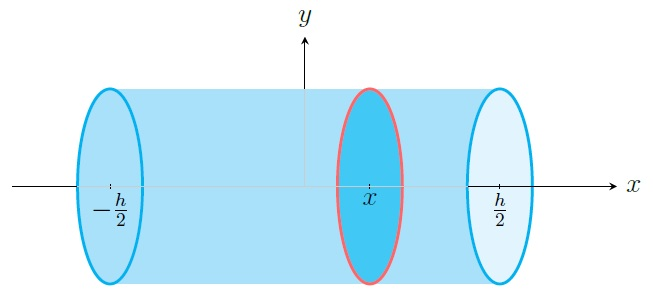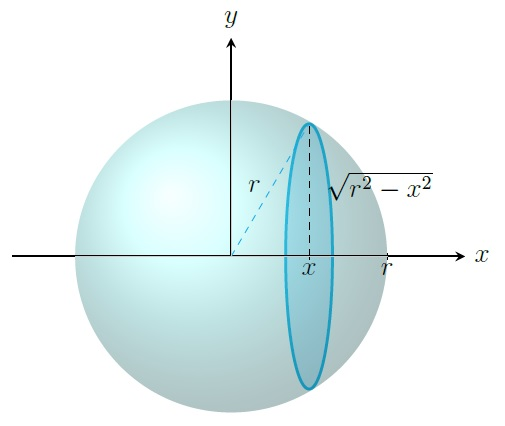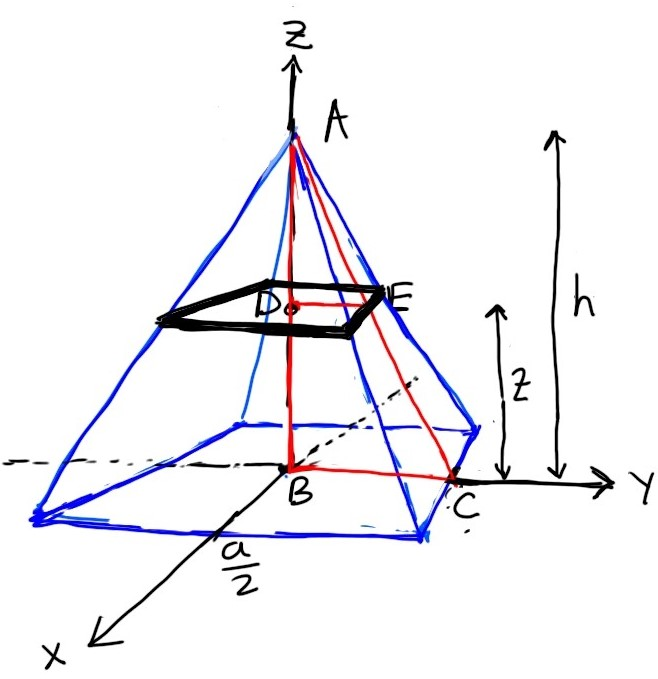Calculus II Home

## Volume of a Solid

Let $$S$$ be the solid between the planes $$x=a$$ and $$x=b$$ such that $$A(x)$$ is the area of its vertical cross-section at $$x$$. If $$A$$ is a continuous function on $$[a,b]$$, then the volume of $$S$$ is $\int_a^b A(x)\;dx.$

Break $$[a,b]$$ into $$n$$ subintervals $$[x_0,x_1],[x_1,x_2],\ldots,[x_{n-1},x_n]$$ where $$x_i=a+i \Delta x$$ and $$\Delta x=(b-a)/n$$. Choose $$x_i^*$$ from $$[x_{i-1},x_i]$$, $$i=1,2,\ldots,n$$. Partition $$S$$ into $$n$$ solids $$S_1,S_2,\ldots,S_n$$ by intersecting $$S$$ by the vertical planes at $$x_1,x_2,\ldots,x_{n-1}$$. Then the volume of $$S_i$$ is approximately $$A(x_i^*) \Delta x$$. Therefore the volume of $$S$$ is $V(S)\approx \sum_{i=1}^n A(x_i^*) \Delta x.$ This approximation of $$V(S)$$ gets better as $$n\to \infty$$. Thus $V(S)=\lim_{n\to \infty} \sum_{i=1}^n A(x_i^*) \Delta x =\int_a^b A(x)\;dx.$

Example. Prove that the volume of a right circular cylinder with radius $$r$$ and height $$h$$ is $$\pi r^2h$$.

Solution. Consider the right circular cylinder with radius $$r$$ that is centered at the origin $$(0,0,0)$$ and lies on $$\left[-\frac{h}{2}, \frac{h}{2} \right]$$ on the $$x$$-axis. Its vertical cross-section at $$x$$ is a disk which has radius $$r$$ and consequently the area $$A(x)=\pi r^2$$. Since the cylinder lies on $$\left[-\frac{h}{2}, \frac{h}{2} \right]$$ on the $$x$$-axis, its volume is $\int_{-\frac{h}{2}}^{\frac{h}{2}} \pi r^2 \;dx= \left. \pi r^2x \right\vert_{-\frac{h}{2}}^{\frac{h}{2}} =\pi r^2\frac{h}{2}-\pi r^2\frac{-h}{2}=\pi r^2h.$Example. Prove that the volume of a sphere of radius $$r$$ is $$\frac{4}{3}\pi r^3$$.

Solution. Consider the sphere of radius $$r$$ that is centered at the origin $$(0,0,0)$$. Its vertical cross-section at $$x$$ is a disk which has radius $$\sqrt{r^2-x^2}$$ and consequently the area $$A(x)=\pi (\sqrt{r^2-x^2})^2=\pi (r^2-x^2)$$. Since the sphere lies on $$[-r,r]$$ on the $$x$$-axis, its volume is $\int_{-r}^r \pi (r^2-x^2) \;dx= \left. \pi\left( r^2x-\frac{x^3}{3} \right) \right\vert_{-r}^r =\pi\left( r^3- \frac{r^3}{3}\right)-\pi\left( -r^3+ \frac{r^3}{3}\right) =\frac{4}{3}\pi r^3.$Example. Prove that the volume of a pyramid of height $$h$$ whose base is a square of side $$a$$ is $$\frac{1}{3}a^2h$$.

Solution. Consider the square pyramid with height $$h$$ whose base is on the $$xy$$-plane centered at the origin $$(0,0,0)$$. Its horizontal cross-section at $$z$$ is square region with side, say $$s$$. By equating the ratio of the base and height of two similar triangles $$\triangle ADE$$ and $$\triangle ABC$$ (see figure), we have $\frac{s/2}{h-z}=\frac{a/2}{h} \implies s=\frac{a(h-z)}{h}=a\left( 1-\frac{z}{h} \right).$ The horizontal cross-section at $$z$$ has the area $A(z)=s^2=a^2\left( 1-\frac{z}{h} \right)^2=a^2\left( 1-2\frac{z}{h}+\frac{z^2}{h^2} \right).$Since the pyramid lies on $$[0,h]$$ on the $$z$$-axis, its volume is $\int_0^h a^2\left( 1-2\frac{z}{h}+\frac{z^2}{h^2} \right) \;dz= \left. a^2\left( z-\frac{z^2}{h}+\frac{z^3}{3h^2} \right) \right\vert_0^h = a^2\left( h-\frac{h^2}{h}+\frac{h^3}{3h^2} \right)=\frac{1}{3}a^2h.$

Last edited